Block
Are you looking to get certified in DevOps, SRE and DevSecOps?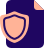DevOps
Get Certified!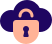SRE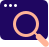DevSecOps
Security is Key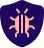Kubernetes
Tomorow's Platform!

# Top 50 Interview Questions and Answers of Keras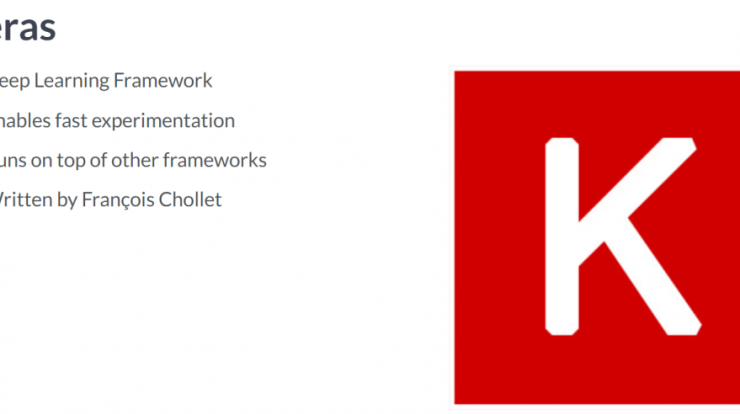Introduction to Keras:

Keras is a high-level neural networks API, capable of running on top of Tensorflow, Theano, and CNTK. It enables fast experimentation through a high-level, user-friendly, modular, and extensible API. Keras can also be run on both CPU and GPU.
Keras was developed and is maintained by Francois Chollet and is part of the Tensorflow core, which makes it Tensorflows preferred high-level API.

Keras is a deep learning API written in Python, running on top of the machine learning platform TensorFlow. It was developed with a focus on enabling fast experimentation. Being able to go from idea to result as fast as possible is key to doing good research.

Keras is the high-level API of TensorFlow 2: an approachable, highly-productive interface for solving machine learning problems, with a focus on modern deep learning. It provides essential abstractions and building blocks for developing and shipping machine learning solutions with high iteration velocity.

Keras empowers engineers and researchers to take full advantage of the scalability and cross-platform capabilities of TensorFlow 2: you can run Keras on TPU or on large clusters of GPUs, and you can export your Keras models to run in the browser or on a mobile device.

let’s move on to Questions and Answers,

1. Who is the Creator of Keras?

Answer: François Chollet, He is currently working as an AI Researcher at Google

2. Types are layers in Keras?

• Core Layers
• Convolutional Layers
• Pooling Layers
• Locally-connected Layers
• Recurrent Layers
• Embedding Layers
• Merge Layers
• Normalization Layers
• Noise layers

3. How can TensorFlow Text be used to preprocess sequence modeling?

4. Explain the examples of data processing in Keras.

Answer: Some of the examples include: Firstly, neural networks don’t process raw data, like text files, encoded JPEG image files, or CSV files. They process vectorized & standardized representations. Secondly, text files need to be read into string tensors, then split into words. Finally, the words need to be indexed and turned into integer tensors. Thirdly, images need to be read and decoded into integer tensors, then converted to floating points and normalized to small values (usually between 0 and 1). Lastly, CSV data needs to be parsed, with numerical features converted to floating-point tensors and categorical features indexed and converted to integer tensors. Then each feature typically needs to be normalized to zero-mean and unit variance.

5. Name the types of inputs in the Keras model.

Answer: Keras models accept three types of inputs: Firstly, NumPy arrays, just like Scikit-Learn and many other Python-based libraries. This is a good option if your data fits in memory. Secondly, TensorFlow Dataset objects. This is a high-performance option that is more suitable for datasets that do not fit in memory and that are streamed from a disk or from a distributed filesystem. Lastly, Python generators that yield batches of data (such as custom subclasses of the keras.utils.Sequence class).

6. Explain the term regularization.

Answer: Regularization is a method that makes slight modifications to the learning algorithm such that the model generalizes better. This in turn improves the model’s performance on the unseen data as well.

7. Name some of the regularization techniques.

The techniques are as follows:

1. L2 and L1 Regularization
2. Dropout
3. Early Stopping
4. Data Augmentation

8. Explain the L2 and L1 Regularization techniques.

Answer: L2 and L1 are the most common types of regularization. Regularization works on the premise that smaller weights lead to simpler models which result helps in avoiding overfitting. So to obtain a smaller weight matrix, these techniques add a ‘regularization term’ along with the loss to obtain the cost function. Here, Cost function = Loss + Regularization term However, the difference between L1 and L2 regularization techniques lies in the nature of this regularization term. In general, the addition of this regularization term causes the values of the weight matrices to reduce, leading to simpler models.

9. What is Convolutional Neural Network?

Answer: A Convolutional Neural Network (ConvNet/CNN) is a Deep Learning algorithm that can take in an input image, assign importance to various aspects/objects in the image and be able to differentiate one from the other. The pre-processing required in a ConvNet is much lower as compared to other classification algorithms. While in primitive methods filters are hand-engineered, with enough training, ConvNets have the ability to learn these filters/characteristics.

10. What do you understand about Dropout and early stopping techniques?

Answer: Dropout means that during the training, randomly selected neurons are turned off or ‘dropped’ out. It means that they are temporarily obstructed from influencing or activating the downward neuron in a forward pass, and none of the weights updates is applied on the backward pass. Whereas Early Stopping is a kind of cross-validation strategy where one part of the training set is used as a validation set, and the performance of the model is gauged against this set. So if the performance on this validation set gets worse, the training on the model is immediately stopped. However, the main idea behind this technique is that while fitting a neural network on training data, consecutively, the model is evaluated on the unseen data or the validation set after each iteration. So if the performance on this validation set is decreasing or remaining the same for certain iterations, then the process of model training is stopped.

11. What do you understand about callbacks?

Answer: Callbacks are an important feature of Keras that is configured in fit(). Callbacks are objects that get called by the model at different points during training like: Firstly, at the beginning and end of each batch Secondly, at the beginning and end of each epoch However, callbacks are a way to make model trainable entirely scriptable. This can be used for periodically saving your model.

12. Explain the process of training a CNN.

Answer: The process for training a CNN for classifying images consists of the following steps −

1. Data Preparation In this step, we center-crop the images and resize them so that all images for training and testing would be of the same size. This is usually done by running a small Python script on the image data.
2. Model Definition In this step, we define a CNN architecture. The configuration is stored in .pb (protobuf) file.
3. Solver Definition In this, we define the solver configuration file. The solver does the model optimization.
4. Model Training In this, we use the built-in Caffe utility to train the model. The training may take a considerable amount of time and CPU usage. After the training is completed, Caffe stores the model in a file, which can, later on, be used on test data and final deployment for predictions.

13. What do you know about Data preprocessing with Keras?

Answer: Once your data is in the form of string/int/float NumpPy arrays, or a Dataset object (or Python generator) that yields batches of string/int/float tensors, it is time to preprocess the data. This can mean: Firstly, Tokenization of string data, followed by token indexing. Secondly, Feature normalization. Thirdly, Rescaling the data to small values. In general, input values to a neural network should be close to zero — typically we expect either data with zero-mean and unit-variance, or data in the [0, 1] range.

14. Explain the process of debugging your model with eager execution.

Answer: If you write custom training steps or custom layers, you will need to debug them. The debugging experience refers to an integral part of a framework and with Keras, the debugging workflow is designed with the user in mind. However, by default, Keras models are compiled to highly optimized computation graphs that deliver fast execution times. That means that the Python code you write is not the code you are actually executing. This introduces a layer of indirection that can make debugging hard. Further, it is better to perform debugging in a step-by-step manner. You want to be able to sprinkle your code with a print() statement to see what your data looks like after every operation, you want to be able to use pdb. You can achieve this by running your model eagerly. With eager execution, the Python code you write is the code that gets executed. Simply pass run_eagerly=True to compile():

15. What is a Keras Tuner?

Answer: Keras Tuner is an easy-to-use, scalable hyperparameter optimization framework that solves the pain points of hyperparameter search. In this, you can easily configure your search space with a define-by-run syntax, then leverage one of the available search algorithms for finding the best hyperparameter values for your models. Further, Keras Tuner comes with Bayesian Optimization, Hyperband, and Random Search algorithms built-in, and is also designed to be easy for researchers to extend in order to experiment with new search algorithms.

16. Explain the role of multiple GPUs in Keras.

Answer: Keras has built-in industry-strength support for multi-GPU training and distributed multi-worker training, via the tf.distribute API. However, if you have multiple GPUs on your machine, you can train your model on all of them by: Firstly, creating a tf.distribute.MirroredStrategy object. Secondly, creating and compiling your model inside the strategy’s scope. Lastly, calling fit() and evaluate() on a dataset as usual.

17. Describe the installation & compatibility of Keras.

Answer: Keras comes packaged with TensorFlow 2 as tensorflow.keras. However, to start using Keras, simply install TensorFlow 2. Keras/TensorFlow is compatible with: Python 3.5–3.8 Ubuntu 16.04 or later Windows 7 or later macOS 10.12.6 (Sierra) or later.

18. What is AutoKeras?

Answer: AutoKeras refers to an AutoML system based on Keras. It is developed by DATA Lab at Texas A&M University. The purpose of AutoKeras is to make machine learning accessible for everyone. It provides high-level end-to-end APIs such as ImageClassifier or TextClassifier to solve machine learning problems in a few lines, as well as flexible building blocks to perform architecture search.

19. How can we create Keras models?

Answer: There are three ways to create Keras models: Firstly, by the Sequential model. This is very straightforward (a simple list of layers), but is limited to single-input, single-output stacks of layers. Secondly using the Functional API. This is an easy-to-use, fully-featured API that supports arbitrary model architectures. This is the Keras “industry strength” model. Lastly, by Model subclassing. Here you implement everything from scratch on your own. Use this if you have complex, out-of-the-box research use cases.

20. Define the following terms: TensorFlow Cloud, TensorFlow.js and TensorFlow Lite.

Answer: TensorFlow Cloud is managed by the Keras team at Google that refers to a set of utilities to help you run large-scale Keras training jobs on GCP with very little configuration effort. Running your experiments on 8 or more GPUs in the cloud should be as easy as calling model.fit(). TensorFlow.js is TensorFlow’s JavaScript runtime, capable of running TensorFlow models in the browser or on a Node.js server, both for training and inference. It natively supports loading Keras models, including the ability to fine-tune or retrain your Keras models directly in the browser. TensorFlow Lite is a runtime for an efficient on-device inference that has native support for Keras models. Deploy your models on Android, iOS, or on embedded devices.

21. Describe the steps for creating a deep Learning Project in Python with Keras.

1. Load Data The first step is for defining the functions and classes. In this, we will use the NumPy library to load our dataset and we will use two classes from the Keras library to define our model.
2. Define Keras Model Models in Keras are defined as a sequence of layers. Here, we will create a Sequential model and add layers one at a time until we are happy with our network architecture. However, ensure that the input layer has the right number of input features. This can be specified when creating the first layer with the input_dim argument and setting it to 8 for the 8 input variables.
3. Compile Keras Model After defining the model, compile it. However, compiling the model uses the efficient numerical libraries under the covers (the so-called backend) such as Theano or TensorFlow. The backend automatically chooses the best way to represent the network for training and making predictions to run on your hardware, such as CPU or GPU or even distributed. During compiling, specify some additional properties required when training the network. And, also specify the loss function to use to evaluate a set of weights, the optimizer is used to search through different weights for the network and any optional metrics we would like to collect and report during training.
4. Fit Keras Model After defining and compiling it is ready for efficient computation. Now, execute the model on some data. We can train or fit our model on our loaded data by calling the fit() function on the model. However, training occurs over epochs and each epoch is split into batches. Epoch: One pass through all of the rows in the training dataset. Batch: One or more samples are considered by the model within an epoch before weights are updated. You must know that one epoch is comprised of one or more batches, depending on the chosen batch size and the model is fit for many epochs.
5. Evaluate Keras Model After training the neural network on the entire dataset and we can examine the performance of the network on the same dataset. However, we can evaluate your model on your training dataset using the evaluate() function on your model and pass it the same input and output used to train the model. Further, the evaluate() function will return a list with two values. The first will be the loss of the model on the dataset and the second will be the accuracy of the model on the dataset.

22. Explain the classes in a Keras Tuner API.

Answer: Firstly, the Hyperparameters class is used for specifying a set of hyperparameters and their values, to be used in the model building function. Secondly, the Tuner subclasses corresponding to different tuning algorithms are called directly by the user to begin the search or to get the best models. Thirdly, the Oracle subclasses are the core search algorithms, receiving model evaluation results from the Tuner and providing new hyperparameter values. Lastly, the HyperModel subclasses are predefined search spaces for certain model families like ResNet and XceptionNet.

23. Define Cross-Validation.

Answer: Cross-validation is a method for examining a machine learning model and testing its performance. CV is mostly used in applied ML tasks. It helps in comparing and selecting an appropriate model for the specific predictive modeling problem. However, it is easy to understand, easy to implement, and it tends to have a lower bias than other methods used to count the model’s efficiency scores.

24. What is Artificial Neural Network?

Answers: Artificial Neural Network (ANN) is at the very core of Deep Learning an advanced version of Machine Learning techniques. ANNs are versatile, adaptive, and scalable, making them appropriate for tackling large datasets and highly complex Machine Learning tasks such as image classification (e.g., Google Images), speech recognition (e.g., Apple’s Siri), video recommendation (e.g., YouTube), or analyzing sentiments among customers (e.g. Twitter Sentiment Analyzer).

25. Describe the algorithm steps used to cross-validate a model.

• At first, divide the dataset into two parts: one for training, other for testing
• Then , training the model on the training set
• Validating the model on the test set
• Lastly, repeating 1-3 steps a couple of times. This number depends on the CV method that you are using

26. Define Cross-Validation in Deep Learning.

Answer: Cross-validation in Deep Learning (DL) can be tricky because most of the CV techniques need training the model at least a couple of times. However, in deep learning, you would normally tempt to avoid CV because of the cost associated with training k different models. Rather than doing k-Fold or other CV techniques, you might use a random subset of your training data as a hold-out for validation purposes. For example, Keras’s deep learning library enables you to pass one of two parameters for the fit function that performs training. This covers: Firstly, validation_split: percentage of the data that should be held out for validation Secondly, validation_data: a tuple of (X, y) which should be used for validation. This parameter overrides the validation_split parameter which means you can use only one of these parameters at once. And, the same method is used in other DL frameworks such as PyTorch and MxNet. They also suggest giving the dataset into three parts: training, validation, and testing.

27. Explain the k-Fold cross-validation technique with the algorithm.

k-Fold CV is a method that minimizes the disadvantages of the hold-out method. k-Fold introduces a new way of splitting the dataset which helps to overcome the “test only once bottleneck”. The algorithm of the k-Fold technique:

• Firstly, select a number of folds – k. Usually, k is 5 or 10 but you can select any number which is less than the dataset’s length.
• Secondly, divide the dataset into k equal (if possible) parts (they are called folds)
• Then, select k – 1 folds which will be the training set. The remaining fold will be the test set
• Fourthly, train the model on the training set. On each iteration of cross-validation, you must train a new model independently of the model trained on the previous iteration
• Then, validate on the test set After that, save the result of the validation
• Now, repeat steps 3 – 6 k times.
• Every time use the remaining fold as the test set.
In the end, you should have validated the model on every fold that you have. Lastly, for having the final score average the results that you got on step 6.

28. Name the types of cross-validation methods.

Some of the cross-validation methods are:

• Hold-out K-folds
• Leave-one-out
• Leave-p-out
• Stratified K-folds
• Repeated K-folds
• Nested K-folds

29. Explain Image Data Augmentation in Keras?

Answer: Image data augmentation is a method that can be used to artificially expand the size of a training dataset by creating modified versions of images in the dataset. However, training deep learning neural network models on more data can result in more skillful models, and the augmentation techniques can create variations of the images that can improve the ability of the fit models to generalize what they have learned to new images. And, the Keras deep learning neural network library provides the capability to fit models using image data augmentation via the ImageDataGenerator class.

30. What is an imbalance classification?

Answer: An imbalanced classification problem is an example of a classification problem in which the distribution of examples over the known classes is biased or skewed. The distribution can range from a slight bias to a severe imbalance where there is one example in the minority class for hundreds, thousands, or millions of examples in the majority class or classes. Further, this creates a challenge for predictive modeling as most of the machine learning algorithms used for classification were designed around the assumption of an equal number of examples for each class. This results in models that have poor predictive performance, specifically for the minority class.

31. Name the main types of data augmentation techniques for image data.

• Image shifts via the width_shift_range and height_shift_range arguments.
• The image flips via the horizontal_flip and vertical_flip arguments.
• Image rotations via the rotation_range argument Image brightness via the brightness_range argument.
• Image zoom via the zoom_range argument.

32. What is a confusion matrix?

Answer: A confusion matrix, also known as an error matrix, is a specific table layout that allows visualization of the performance of an algorithm, typically a supervised learning one. Every row of the matrix represents the instances in an actual class while each column represents the instances in a predicted class, or vice versa. The name stems from the fact that it makes it easy to see whether the system is confusing two classes. Further, it can be considered as a special kind of contingency table, with two dimensions (“actual” and “predicted”), and identical sets of “classes” in both dimensions.

33. Why is there a need for keras?

Answer: Keras is an API designed for human beings, not machines. Keras follows best practices for reducing cognitive load: it offers consistent & simple APIs, it minimizes the number of user actions required for common use cases, and it provides clear and actionable feedback upon user error.

34. What is flatten layer in keras?

Answer: The role of the Flatten layer in Keras is super simple: A flatten operation on a tensor reshapes the tensor to have a shape that is equal to the number of elements contained in the tensor.

35. Is keras a library?

Answer: Yes, Keras is an open-source neural-network library written in Python.

36. What is keras dropout?

Answer: Dropout is a regularization technique for neural network models proposed by Srivastava, it is a technique where randomly selected neurons are ignored during training.

37. What are regularizers in keras?

keras.regularizers.l1(0.)
keras.regularizers.l2(0.)
keras.regularizers.l1_l2(l1=0.01, l2=0.01)

38. What are available constraints in keras?

• MaxNorm
• NonNeg
• UnitNorm
• MinMaxNorm

39. How can I use Keras with datasets that don’t fit in memory?

You should use the tf.data API to create tf.data.Dataset objects — an abstraction over a data pipeline that can pull data from local disk, from a distributed file system, from GCS, etc., as well as efficiently apply various data transformations.

For instance, the utility tf.keras.preprocessing.image_dataset_from_directory will create a dataset that reads image data from a local directory. Likewise, the utility tf.keras.preprocessing.text_dataset_from_directory will create a dataset that reads text files from a local directory.

Dataset objects can be directly passed to fit(), or can be iterated over in a custom low-level training loop.

model.fit(dataset, epochs=10, validation_data=val_dataset)

40. What is a Neural Network?

Answer: Neural Networks replicate the way humans learn, inspired by how the neurons in our brains fire, only much simpler.

41. What Is a Multi-layer Perceptron(MLP)?

As in Neural Networks, MLPs have an input layer, a hidden layer, and an output layer. It has the same structure as a single layer perceptron with one or more hidden layers. A single layer perceptron can classify only linear separable classes with binary output (0,1), but MLP can classify nonlinear classes.

Except for the input layer, each node in the other layers uses a nonlinear activation function. This means the input layers, the data coming in, and the activation function is based upon all nodes and weights being added together, producing the output. MLP uses a supervised learning method called “backpropagation.” In backpropagation, the neural network calculates the error with the help of cost function. It propagates this error backward from where it came (adjusts the weights to train the model more accurately).

42. What Is the Role of Activation Functions in a Neural Network?

Answer: At the most basic level, an activation function decides whether a neuron should be fired or not. It accepts the weighted sum of the inputs and bias as input to any activation function. Step function, Sigmoid, ReLU, Tanh, and Softmax are examples of activation functions.

43. What Are the Applications of a Recurrent Neural Network (RNN)?

Answer: The RNN can be used for sentiment analysis, text mining, and image captioning. Recurrent Neural Networks can also address time series problems such as predicting the prices of stocks in a month or quarter.

44. What Are Hyperparameters?

45. What Is the Difference Between Batch Gradient Descent and Stochastic Gradient Descent?

46. What is Overfitting and Underfitting, and How to Combat Them?

Overfitting occurs when the model learns the details and noise in the training data to the degree that it adversely impacts the execution of the model on new information. It is more likely to occur with nonlinear models that have more flexibility when learning a target function. An example would be if a model is looking at cars and trucks, but only recognizes trucks that have a specific box shape. It might not be able to notice a flatbed truck because there’s only a particular kind of truck it saw in training. The model performs well on training data, but not in the real world.

Underfitting alludes to a model that is neither well-trained on data nor can generalize to new information. This usually happens when there is less and incorrect data to train a model. Underfitting has both poor performance and accuracy.

To combat overfitting and underfitting, you can resample the data to estimate the model accuracy (k-fold cross-validation) and by having a validation dataset to evaluate the model.

47. How Are Weights Initialized in a Network?

Answer: There are two methods here: we can either initialize the weights to zero or assign them randomly.

Initializing all weights to 0: This makes your model similar to a linear model. All the neurons and every layer perform the same operation, giving the same output and making the deep net useless.

Initializing all weights randomly: Here, the weights are assigned randomly by initializing them very close to 0. It gives better accuracy to the model since every neuron performs different computations. This is the most commonly used method.

48. What is Pooling on CNN, and How Does It Work?

Answer: Pooling is used to reduce the spatial dimensions of a CNN. It performs down-sampling operations to reduce the dimensionality and creates a pooled feature map by sliding a filter matrix over the input matrix.

49. What Is the Difference Between Epoch, Batch, and Iteration in Deep Learning?

• Epoch – Represents one iteration over the entire dataset (everything put into the training model).
• Batch – Refers to when we cannot pass the entire dataset into the neural network at once, so we divide the dataset into several batches.
• Iteration – if we have 10,000 images as data and a batch size of 200. then an epoch should run 50 iterations (10,000 divided by 50).

50. Why is Tensorflow the Most Preferred Library in Deep Learning?

Answer: Tensorflow provides both C++ and Python APIs, making it easier to work on and has a faster compilation time compared to other Deep Learning libraries like Keras and Torch. Tensorflow supports both CPU and GPU computing devices.